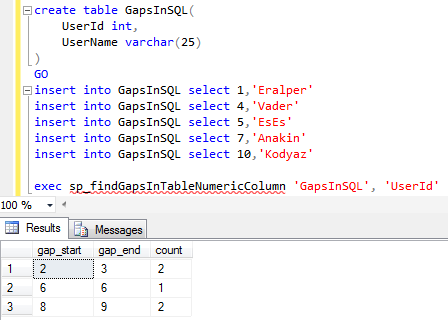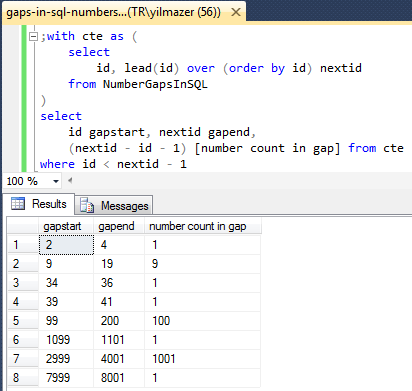SQL Server and T-SQL Development Tutorials Development resources, articles, tutorials, code samples and tools and downloads for ASP.Net, SQL Server, R Script, Windows, Windows Phone, AWS, SAP HANA and ABAP, like SAP UI5, Screen Personas, etc.
 Home Articles News IT Jobs Tools Sample Chapters Trainers Blogs Forums Photos Files

SQL Server 2019 Installation

# Use SQL to Find Missing Numbers and Gaps in Sequence of Numbers like Identity Column

SQL developers are asked to use SQL to find missing numbers in a sequence column or find sequence gaps in numbers like the gaps in an identity column of a SQL Server database table. Although it is easy to list missing numbers within a given number sequence using a numbers table with Left Join, to identify number gaps by identifying the lower bound and upper bound of the gaps may be difficult for many SQL developers.

In fact, finding gaps in a number sequence is one of the most popular SQL programming problem for SQL developers.
The first solution to define gaps in numbers can be used for SQL Server 2008 R2 and previous versions including (SQL Server 2005) where Row_Number() and aggregate functions with Partition By clause are used.
And the second method which is more simple to find gaps in number sequence is using SQL LEAD() function which is introduced with SQL Server 2012 for Transact-SQL developers.

In this SQL tutorial I want to share SQL source codes for a generic solution that can be applied to many cases to find missing numbers and identify number gaps in sequence as well as continuous number ranges and numeric islands in a sequence with both upper and lower bounds.## Find Missing Numbers and Gaps in a Sequence of Numbers

Let's start our SQL tutorial for finding number gaps with a SQL script to create a sample database table and populate it with sample data.

Below Create Table command creates a database table named NumberGapsInSQL with an identity column and an additional text column. Our task will be identifying the gaps in identity column in our sample table.

``` create table NumberGapsInSQL (  id int identity(1,1),  number varchar(20) ) ```

After table is created in the SQL Server database, we are now ready to pupulate it with test data. I'll use a loop using SQL WHILE command and a counter to insert 10000 rows into the test table.

``` declare @i int set @i = 1 while @i <= 10000 begin  insert into NumberGapsInSQL    values ('Number is ' + cast(@i as varchar(10)))  set @i = @i + 1 end ```

After sql database table is populated with sample data, we can now simulate a real life case where some of the inserted rows are deleted with time.

First SQL Delete command removes specific rows with given IDs specified using IN clause.
Following Delete statements removes id ranges which create gaps in the number series.

``` delete NumberGapsInSQL where id in (3, 35, 40, 1100, 8000) delete NumberGapsInSQL where id between 10 and 18 delete NumberGapsInSQL where id between 100 and 199 delete NumberGapsInSQL where id between 3000 and 4000 ```

SQL programmers should be able to identify the single IDs as well as ID gaps missing in the Identity column of the sample database table.

First of all, I use a numbers table to identify missing numbers in the series which create the number gaps. I used the dbo.NumbersTable SQL numbers table function using SQL recursive CTE (Common Table Expression) query.

The numbers table should be able to return values starting from 1 up to the maximum ID number in the target database table. SQL developers can execute "select max(id) from NumberGapsInSQL" SQL Select command to return the maximum number in the numeric table column.
I can use this value as an argument to the dbo.NumbersTable() user defined table function as follows:

``` select * from dbo.NumbersTable(1,(select max(id) from NumberGapsInSQL),1) ```

When you execute the above SELECT statement, developers will see that they have a list of all integers between the range 1 and maximum number stored in the target table identity column

If we join numbers table and target NumberGapsInSQL table using a LEFT OUTER JOIN and apply a filter on ID column of the target table as follows, we will be able to list all missing numbers in the related numeric column.

``` select n.i from dbo.NumbersTable(1,(select max(id) from NumberGapsInSQL),1) n left join NumberGapsInSQL g on g.id = n.i where g.id is null order by n.i ```

Of course, some Transact-SQL developers can think that this SQL Select statement is good enough to get the missing values in the numeric column. And some developers will not be happy with the 1115 rows just giving the integer numbers of deleted rows' identity values but not the gaps identified with their lower and upper bounds.

I'm one of the SQL developers that are not happy with a list without a shorter gap describing outcome.
So I worked on the below sql script which can return the list of numeric value gaps in the sample data.

## Find Gaps in Sequence Numbers using SQL

Since this number gap detect SQL script uses ROW_NUMBER() function and MIN() and MAX() functions with PARTITION BY clause, SQL developers and database administrators can apply the below solution on SQL Server 2005 and later versions. As I mentioned before if you are using SQL Server 2012 and SQL Server 2014 (Hekaton), then SQL developers can use new SQL Lead function as demonstrated in the next solution.

``` ;with missing as (  SELECT   n.i  FROM dbo.NumbersTable(1,(select max(id) from NumberGapsInSQL),1) n  LEFT JOIN NumberGapsInSQL g ON g.id = n.i  WHERE g.id is null ), sorted as (  select   rn = ROW_NUMBER() over (order by i),   i  from missing ), gap_start as (  select   i,   min(i) over (partition by i-rn order by i) as gap_start  from sorted ), gap_end as (  select   gap_start,   max(i) over (partition by gap_start order by i desc) as gap_end  from gap_start ) select distinct *, (1+gap_end-gap_start) count from gap_end ```

When above SQL CTE expression and Select statement is executed to find missing numbers and to find gaps in the sequence of identity column values, below result set is returned by the SQL Server engine.
Each gap or hole in the identity column values is listed as a seperate row. Each row (representing a gap or a sequence of missing values) is represented with lower bound and upper bound values as well as the count of missing integers in the sequence gap.Above SQL solution can be applied as a generic solution to find gaps in number columns which is one of the most common problems for database developers. To make the above SQL script appliable to every case, I created a dynamic SQL command within a stored procedure and passed the table name and column name as arguments to this SQL stored procedure

At the end of the stored procedure, developers will see that "EXEC sp_ExecuteSQL" command line is used to run the dynamic SQL statement and return the identified gaps as a list with min and max values of the gaps.

``` create procedure sp_findGapsInTableNumericColumn(   @tablename sysname,   @columnname sysname ) as declare @SQL nvarchar(max) set @SQL = ' ;with missing as (  SELECT   n.i  FROM dbo.NumbersTable(1,(select max(' + @columnname + ') from ' + @tablename + '),1) n  LEFT JOIN ' + @tablename + ' g ON g.' + @columnname + ' = n.i  WHERE g.' + @columnname + ' is null ), sorted as (  select   rn = ROW_NUMBER() over (order by i),   i  from missing ), gap_start as (  select   i,   min(i) over (partition by i-rn order by i) as gap_start  from sorted ), gap_end as (  select   gap_start,   max(i) over (partition by gap_start order by i desc) as gap_end  from gap_start ) select distinct *, (1+gap_end-gap_start) count from gap_end' exec sp_executeSQL @sql go -- Sample usage for finding gaps in numeric column ColumnName of table TableName --exec sp_findGapsInTableNumericColumn 'TableName', 'ColumnName' ```

Here is a sample case where I used this new SQL stored procedure to demonstrate how can developers use it to list number gaps in identity column of a database table using SQL.I hope above SQL codes to find gaps in numbers within a SQL Server database will be helpful for database application developers and DBAs which are working on versions prior SQL Server 2012.

## List Gaps in Numbers Sequence on SQL Server 2014

If SQL programmers consider the database performance, then they can apply SQL Lead() function solution since following SQL script is the fastest code to detect and list gaps in a numbers sequence. Unfortunately since SQL Lead() analytic function is an enhancement introduced first with SQL Server 2012, database developers can apply Lead function for developments on SQL Server 2012 and SQL Server 2014 (Hekaton) at the moment.

Below SQL code is easy to read for database programmers which are familiar with Lead() analytic function. Lead() function column returns the next id in a sorted sequence as defined with Order By clause. So in the CTE (Common Table Expression), we have selected id and next id in the number sequence. If there is difference more than 1 between these two columns, this means that a gap exists between id and next id fetched with SQL Lead() function.

``` ;with cte as (  select   id, lead(id) over (order by id) nextid  from NumberGapsInSQL ) select  id gapstart, nextid gapend,  (nextid - id - 1) [number count in gap] from cte where id < nextid - 1 ```

Here is the output of the above script.As database administrators and programmers will realize, this script is easier to read and understand and this gap detect code performs better than the first solution introduced in this SQL tutorial.

Here is a stored procedure which detects gaps on a table column values. The stored procedure codes use the new SQL Lead() function as explained above.

``` create procedure sp_findGapsInTableNumericColumn(  @tablename sysname,  @columnname sysname ) as declare @SQL nvarchar(max) set @SQL = ' with cte as ( select ' + @columnname + ' id, lead(' + @columnname + ') over (order by ' + @columnname + ') nextid from ' + @tablename + ' ) select id gapstart, nextid gapend, (nextid - id - 1) [number count in gap] from cte where id < nextid - 1' exec sp_executeSQL @sql go -- Detect numberic gaps using sp_findGapsInTableNumericColumn exec sp_findGapsInTableNumericColumn 'NumberGapsInSQL', 'Id' ```

Related SQL Resources

SQL Server Articles

SQL Server 2012

SQL Server Tools

SQL Blog

MS SQL Server Forums